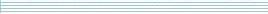| home      | people     | research     | publications    | seminars    | events     | contactCommunication Networks   | Systems Biology   | Hybrid Systems    | Machine Learning   | Dynamics & Interaction### Schools Mathematics Grand Challenge

First Week's Puzzles

Problem 1:

The first problem was:

As I was going to St. Ives
I met a man with seven wives,
Each wife had seven sacks,
Each sack had seven cats,
Each cat had seven kits:

kits, cats, sacks and wives,
How many, in total, did I meet while going to St. Ives?

(You need to give the grand total (one number) of kits+cats+sacks+wives)

Solution:

You were asked for the total number of kits, cats, sacks and wives.
Wives: Total number of wives = 7
Sacks: Each wife has 7 so total number of sacks = 7x7 = 7^2
Cats: Each sack has 7 cats so total number of cats = 7x7x7 = 7^3
Kits: Again, each cat has 7 kits so total number of kits = 7x7x7x7 = 7^4

Hence the grand total of kits+cats+sacks+wives is 7+7^2+7^3+7^4 = 2800.

Problem 2:

The second problem was:

Fifteen years ago, my mother's age was three times my age. She is now twice my age. What age am I now?

Solution:

Let X be my age and Y be my mother's age. We have two pieces of information, both of which we should use.

My mothers age is twice my age. This means that

Y = 2X

15 years ago my mother's age was three times my age. This means that

Y - 15 = 3(X - 15)

But Y = 2X so

2X - 15 = 3X - 45

and

X = 30.

So, my age is thirty.